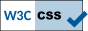ESSLLI 2011 tutorial: Automated Synthesis of Tableau Calculi
Lecturers: Dr R. A. Schmidt and Dr D. Tishkovsky

## Course Description

Non-classical logics, including modal logics, description logic, hybrid logics and intuitionistic logic, are popular and have many applications. The applications range from multi-agent systems to medical domains, web services and the semantic web. Demand for deduction calculi and implemented provers for non-classical logics is thus high. Rather than developing deduction calculi one by one and proving important properties such as soundness, completeness and decidability for each individually, in this course we discuss the possibility of providing a generic framework for developing deduction calculi. The idea is that deduction calculi can be developed for all these different logics and their applications in a uniform way. There are various interactive prover engineering platform that can be used for developing calculi. These provide flexible and general frameworks for defining and experimenting with implementations of different sets of tableau inference rules for different logics, different rule application strategies and different optimisations.

As an alternative, in this course we present a framework for fully-automatically generating deduction calculi from the specification of a logic. The logic of interest is assumed to be defined by a high-level specification of its formal semantics. The aim is to turn this into a set of inference rules, which forms a sound and complete deduction calculus for the logic. Ideally we also want to be able to guarantee termination if the logic is decidable. Automated synthesis of calculi is a very challenging problem and in general it is of course not possible to turn every specification of a logic into a sound, complete and terminating deduction calculus. It is however possible to solve the problem for a large number of logics.

This course will focus on the tableau calculus synthesis framework we introduced earlier. The type of calculi generated are ground semantic tableau calculi that operate on labelled formulae where the labels represent individuals or states in the underlying models.There were 48037 visits of this page since Wed, 01 Jun 2011 00:00:00 BST. Last modified: Wed, 01 Jun 2011 22:10:40 BST. Copyright ©2011 Dmitry Tishkovsky, School of Computer Science, University of Manchester